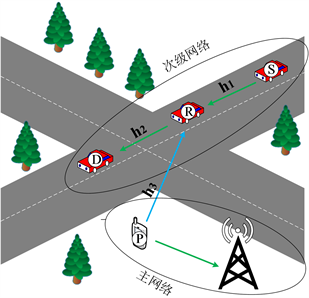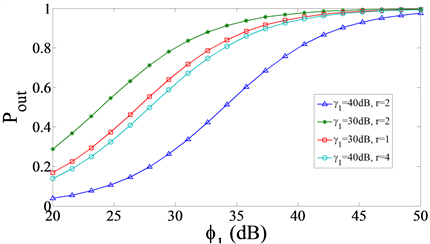﻿ 认知无线电协同传输在车联网中的应用及性能分析

# 认知无线电协同传输在车联网中的应用及性能分析Application and Performance analysis of Cognitive Radio Cooperative Transmission in Internet of Vehicles

Abstract: With the rapid development of communication and smart antenna technology, the Internet of Vehicles (IoV) has received extensive attention and research. The application of IoV is hampered by lack of spectrum and limited communication coverage. In this paper, cognitive radio cooperative transmission is introduced into the IoV system to expand the transmission range without increasing the transmission power. The outage probability of end-to-end transmission is calculated by analyzing and deducing the signal to interference plus noise ratio. Numerical simulation results show that compared with direct communication, the use of cognitive radio cooperative transmission can effectively reduce the outage probability and improve the performance of the IoV.

1. 引言

 将最佳中继协同传输引入到认知无线电中，推导了对主网络的干扰小于或等于阈值的情况下，精准的中断概率表达式。但  中的协同中继转发策略依赖大量信道状态信息，实现起来并没有那么容易。在  中提出来一种新的中继协同传输方法，通过选择部分具有固定增益的中继，减少对于信道信息的需求。   都为单中继协同传输策略，  提出了多中继的协同传输策略，它们以牺牲一定的带宽来获得更高的信干噪比。

2. 系统模型及信干噪比分析

2.1. 系统模型Figure 1. System model

2.2. 信干噪比分析

${y}_{1}=\sqrt{{P}_{1}}{h}_{1}{x}_{1}+{n}_{0}+\sqrt{{P}_{3}}{h}_{3}{x}_{2}$ (1)

${y}_{2}=\sqrt{{P}_{2}}{h}_{2}{x}_{1}+{n}_{0}$ (2)

${\zeta }_{1}=\frac{{P}_{1}{|{h}_{1}|}^{2}}{{N}_{0}+{P}_{3}{|{h}_{3}|}^{2}}=\frac{\frac{{P}_{1}{|{h}_{1}|}^{2}}{{N}_{0}}}{1+\frac{{P}_{3}{|{h}_{3}|}^{2}}{{N}_{0}}}=\frac{{\gamma }_{1}}{1+{\varphi }_{1}}$ (3)

${\zeta }_{2}=\frac{{P}_{2}{|{h}_{2}|}^{2}}{{N}_{0}}={\gamma }_{2}$ (4)

$x\ge 1$ 时， ${F}_{{\varphi }_{1}+1}\left(x\right)=\mathrm{Pr}\left\{{\varphi }_{1}+1\le x\right\}=\mathrm{Pr}\left\{{\varphi }_{1}\le x-1\right\}={F}_{{\varphi }_{1}}\left(x-1\right)$${\varphi }_{1}+1$ 分布函数为：

${F}_{{\varphi }_{1}+1}\left(x\right)=1-{\text{e}}^{-{\mu }_{3}\left(x-1\right)}$ (5)

${f}_{{\varphi }_{1}+1}\left(x\right)={{F}^{\prime }}_{{\varphi }_{1}+1}\left(x\right)={\mu }_{3}{\text{e}}^{-{\mu }_{3}\left(x-1\right)}$ (6)

$x<1$ 时， ${F}_{{\varphi }_{1}+1}\left(x\right)=0$${f}_{{\varphi }_{1}+1}\left(x\right)=0$

$\begin{array}{l}\because {F}_{{\zeta }_{1}}\left(x\right)=\mathrm{Pr}\left\{\frac{{\gamma }_{1}}{1+{\varphi }_{1}}\le x\right\}={\int }_{0}^{\infty }\mathrm{Pr}\left\{{\gamma }_{1}\le xt\right\}\cdot {f}_{{\varphi }_{1}+1}\left(t\right)\text{d}t\\ \text{\hspace{0.17em}}\text{\hspace{0.17em}}\text{\hspace{0.17em}}\text{\hspace{0.17em}}\text{\hspace{0.17em}}\text{\hspace{0.17em}}\text{\hspace{0.17em}}\text{\hspace{0.17em}}\text{\hspace{0.17em}}\text{\hspace{0.17em}}\text{\hspace{0.17em}}\text{\hspace{0.17em}}={\int }_{0}^{\infty }\left(1-{\text{e}}^{-{\mu }_{1}\left(xt\right)}\right)\cdot \left({\mu }_{3}{\text{e}}^{-{\mu }_{3}\left(t-1\right)}\right)\text{d}t={\text{e}}^{{\mu }_{3}}-\frac{{\mu }_{3}{\text{e}}^{{\mu }_{3}}}{{\mu }_{1}x+{\mu }_{3}}\end{array}$ (7)

(7)式为车辆S到车辆R链路的信干噪比分布函数，又因为 ${\zeta }_{2}=\frac{{P}_{2}{|{h}_{1}|}^{2}}{{N}_{0}}={\gamma }_{2}~\mathrm{exp}\left({\mu }_{2}\right)$

${\zeta }_{2}$ 的分布函数为：

${F}_{{\zeta }_{2}}\left(x\right)=1-{\text{e}}^{-{\mu }_{2}x}$ (8)

${\zeta }_{e2e}=\mathrm{min}\left\{{\zeta }_{1},{\zeta }_{2}\right\}$

$\begin{array}{c}{F}_{{\zeta }_{e2e}}\left(x\right)=\mathrm{Pr}\left\{\mathrm{min}\left\{{\zeta }_{1},{\zeta }_{2}\right\}\le x\right\}=1-\mathrm{Pr}\left\{\mathrm{min}\left\{{\zeta }_{1},{\zeta }_{2}\right\}>x\right\}\\ =1-\mathrm{Pr}\left\{{\zeta }_{1}>x\right\}\cdot \mathrm{Pr}\left\{{\zeta }_{2}>x\right\}=1-\left(1-{F}_{{\zeta }_{1}}\left(x\right)\right)\cdot \left(1-{F}_{{\zeta }_{2}}\left(x\right)\right)\end{array}$ (9)

${F}_{{\zeta }_{e2e}}\left(x\right)=1-\left(1-{\text{e}}^{{\mu }_{3}}+\frac{{\mu }_{3}{\text{e}}^{{\mu }_{3}}}{{\mu }_{1}x+{\mu }_{3}}\right)\cdot \left({\text{e}}^{-{\mu }_{2}x}\right)$ (10)

3. 性能分析及数值模拟

3.1. 中断概率

${P}_{out}=\mathrm{Pr}\left(\frac{1}{2}{\mathrm{log}}_{2}\left(1+{\zeta }_{e2e}\right)\le r\right)={F}_{{\zeta }_{e2e}}\left({2}^{2r}-1\right)$

${P}_{out}=1-\left(1-{\text{e}}^{{\mu }_{3}}+\frac{{\mu }_{3}{\text{e}}^{{\mu }_{3}}}{{\mu }_{1}\left({2}^{2r-1}\right)+{\mu }_{3}}\right)\cdot \left({\text{e}}^{-{\mu }_{2}\left({2}^{2r-1}\right)}\right)$ (11)

3.2. 数值模拟Figure 2. The influence curve of ${\gamma }_{1}$ on ${P}_{out}$Figure 3. The influence curve of ${\varphi }_{1}$ on ${P}_{out}$

4. 结论

 Haykin, S. (2005) Cognitive Radio: Brain-Empowered Wireless Communications. IEEE Journal on Selected Areas in Communications, 23, 201-220.
https://doi.org/10.1109/JSAC.2004.839380

 曹华孝, 鲜永菊, 徐昌彪. 认知无线电技术的国内外发展与研究现状[J]. 数字通信, 2009, 36(2): 62-68.

 刘元, 彭端, 陈楚. 认知无线电的关键技术和应用研究[J]. 通信技术, 2007, 40(7): 87-90.

 Kusaladharma, S. and Tellambura, C. (2012) Aggregate Interference Analysis for Underlay Cognitive Radio Networks. IEEE Wireless Communication Letters, 1, 641-644.
https://doi.org/10.1109/WCL.2012.091312.120600

 Suoping, L., Wei, L., Jaafar, G., Kejun, J. and Fan, W. (2020) Design and Analysis of Adaptive Full-Duplex Cognitive Relay Cooperative Strategy Based on Primary System Behavior. Wireless Networks, 26, 6237-6252.
https://doi.org/10.1007/s11276-020-02433-w

 Zou, Y., Zhu, J., Zheng, B. and Yao, Y. (2010) An Adaptive Cooperation Diversity Scheme with Best-Relay Selection in Cognitive Radio Networks. IEEE Transactions on Signal Processing, 58, 5438-5445.
https://doi.org/10.1109/TSP.2010.2053708

 Simeone, O., Bar-Ness, Y. and Spagnolini, U. (2007) Stable Throughput of Cognitive Radios with and without Relaying Capability. IEEE Transactions on Communications, 55, 2351-2360.
https://doi.org/10.1109/TCOMM.2007.910699

 Ashrafinia, S., Pareek, U. and Naeem, M. (2011) Biogeography-Based Optimization for Joint Relay Assignment and Power Allocation in Cognitive Radio System. 2011 IEEE Symposium on Swarm Intelligence, Paris, 11-15 April 2011, 1-8.
https://doi.org/10.1109/SIS.2011.5952589

 Elalem, M., Zhao, L. and Liao, Z. (2010) Interference Mitigation Using Power Control in Cognitive Radio Networks. Vehicular Technology Conference, Taipei, 16-19 May 2010, 1-5.
https://doi.org/10.1109/VETECS.2010.5494050

 Anghel, P.A. and Kaveh, M. (2004) Exact Symbol Error Probability of a Cooperative Network in a Rayleigh-Fading Environment. IEEE Transactions on Wireless Communications, 3, 1416-1421.
https://doi.org/10.1109/TWC.2004.833431

 Yu, S., Lee, J., Park, K., Das, A.K. and Park, Y. (2020) IoV-SMAP: Secure and Efficient Message Authentication Protocol for IoV in Smart City Environment. IEEE Access, 8, 167875-167886.
https://doi.org/10.1109/ACCESS.2020.3022778

 程刚, 郭达. 车联网现状与发展研究[J]. 移动通信, 2011, 35(17): 23-26.

 Li, S.P., Wang, F., Gaber, J. and Chang, X.K. (2020) Throughput and Energy Efficiency of Cooperative ARQ Strategies for VANETs Based on Hybrid Vehicle Communication Mode. IEEE Access, 8, 114287-114304.
https://doi.org/10.1109/ACCESS.2020.3003813

 Islam, S.N., Sadeghi, P. and Durrani, S. (2013) Error Performance Analysis of Decode-and-Forward and Amplify-and-Forward Multi-Way Relay Networks with Binary Phase Shift Keying Modulation. IET Communications, 7, 1605-1616.
https://doi.org/10.1049/iet-com.2013.0284

 Ikki, S. and Ahmed, M.H. (2007) Performance Analysis of Cooperative Diversity Wireless Networks over Nakagami-m Fading Channel. IEEE Communications Letters, 11, 334-336.
https://doi.org/10.1109/LCOM.2007.348292

Top